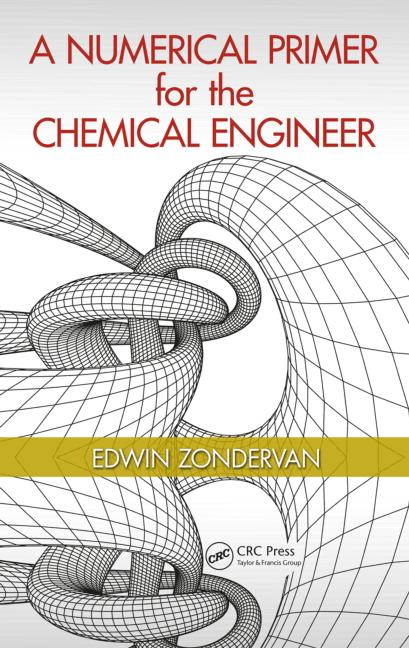A Numerical Primer for the Chemical Engineer

1st Edition

Edwin Zondervan

CRC Press
Published August 12, 2014
Textbook - 193 Pages - 61 B/W Illustrations
ISBN 9781482229448 - CAT# K22617

For Instructors Request Inspection Copy

was \$94.95

USD\$75.96

SAVE ~\$18.99

FREE Standard Shipping!

Preview

Summary

Solve Developed Models in a Numerical Fashion

Designed as an introduction to numerical methods for students, A Numerical Primer for the Chemical Engineer explores the role of models in chemical engineering. Combining mathematical correctness (model verification) with numerical performance (model validation), this text concentrates on numerical methods and problem solving, rather than focusing on in-depth numerical analysis. It applies actual numerical solution strategies to formulated process models to help identify and solve chemical engineering problems.

Describe Motions with Accuracy

The book starts with a recap on linear algebra, and uses algorithms to solve linear equations, nonlinear equations, ordinary differential equations, and partial differential equations (PDEs). It includes an introductory chapter on MATLAB® basics, contains a chapter on the implementation of numerical methods in Excel, and even adopts MATLAB and Excel as the programming environments throughout the text.

The material addresses implicit and explicit schemes, and explores finite difference and finite volume methods for solving transport PDEs. It covers the methods for error and computational stability, as well as curve fitting and optimization. It also contains a case study chapter with worked out examples to demonstrate the numerical techniques, and exercises at the end of each chapter that students can use to familiarize themselves with the numerical methods.

A Numerical Primer for the Chemical Engineer lays down a foundation for numerical problem solving and sets up a basis for more in-depth modeling theory and applications. This text addresses the needs of senior undergraduates in chemical engineering, and students in applied chemistry and biochemical process engineering/food process engineering.

Instructors

We provide complimentary e-inspection copies of primary textbooks to instructors considering our books for course adoption.